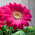### Queue implementation using Linked List

Queue is a data structure that serves as a collection of elements. It follows the policy of FIFO i.e first in first out. To follow this it has 2 principle functions

1. Enqueue  function is used to add an element to the rear of the queue
2. Dequeue function is used to remove an element from the queue

Linked list is a simple linear data structure formed by collection of data elements called nodes. Each node consists of a data element and link field.The data field consists of element and link field consists of address of next element.

Now inorder to implement Queue using linked list we need to implement enqueue and dequeue operation in Linked List. Enqueue and dequeue can be implemented by using other already implemented functions of Linked List like addToEnd and remove functions.

Here is the Complete code for the implementation

### C++ Program

You might Also be interested in

Binary Search Tree
Height of Binary Search Tree

1.### Infix to Prefix conversion using Stack

This post is about conversion of Infix expression to Prefix conversion. For this conversion we take help of stack data structure, we need to push and pop the operators in and out of the stack.

Infix expressions are the expressions that we normally use,eg. 5+6-7; a+b*c etc. Prefix expressions are the expressions in which the 2 operands are preceded by the operator eg. -+567 , +a*bc etc.

This method is very similar to the method that we used to convert Infix to Postfix but the only difference is that here we need to reverse the input string before conversion and then reverse the final output string before displaying it.

NOTE: This changes one thing that is instead of encountering the opening bracket we now first encounter the closing bracket and we make changes accordingly in our code.

So, to convert an infix expression to a prefix expression we follow the below steps
(we have 2 string, 1st is the input infix expression string 2nd is the output string which is empty initially)

We first revers…

Hashing is a technique used for storing , searching and removing elements in almost constant time. Hashing is done with help of a hash function that generates index for a given input, then this index can be used to search the elements, store an element, or remove that element from that index.

A hash function is a function that is used to map the data elements to their position in the data structure used. For example if we use an array to store the integer elements then the hash function will generate position for each element so that searching, storing and removing operation on the array can be done in constant time that is independent of the number of elements in the array. For better look at the example below.

now we face a problem if for 2 numbers same position is generated example consider elements 1 and 14

1 % 13 = 1

14 % 13 = 1

so when we get 1 we store it at the first position, but when we get 14 we see that the position 1 is already taken, this is a case of collision.

Inorder…